properties of light that ray theory is unable to explain. The mode theory uses electromagnetic wave behavior to describe the propagation of light along a fiber. A set of guided electromagnetic waves is called the modes of the fiber. "> Mode TheoryCustom SearchMode Theory The mode theory, along with the ray theory, is used to describe the propagation of light along an optical fiber. The mode theory is used to describe the properties of light that ray theory is unable to explain. The mode theory uses electromagnetic wave behavior to describe the propagation of light along a fiber. A set of guided electromagnetic waves is called the modes of the fiber. Q.26 The mode theory uses electromagnetic wave behavior to describe the propagation of the light along the fiber. What is a set of guided electromagnetic waves called? PLANE WAVES. - The mode theory suggests that a light wave can be represented as a plane wave. A plane wave is described by its direction, amplitude, and wavelength of propagation. A plane wave is a wave whose surfaces of constant phase are infinite parallel planes normal to the direction of propagation. The planes having the same phase are called the wavefronts. The wavelength (λ) of the plane wave is given by:where c is the speed of light in a vacuum, f is the frequency of the light, and n is the index of refraction of the plane-wave medium. Figure 2-14 shows the direction and wavefronts of plane-wave propagation. Plane waves, or wavefronts, propagate along the fiber similar to light rays. However, not all wavefronts incident on the fiber at angles less than or equal to the critical angle of light acceptance propagate along the fiber. Wavefronts may undergo a change in phase that prevents the successful transfer of light along the fiber. Figure 2-14. - Plane-wave propagation.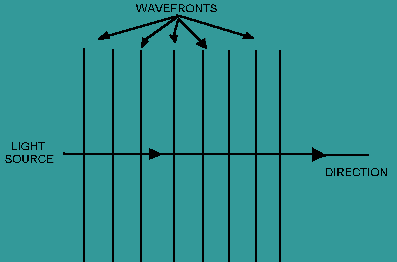Wavefronts are required to remain in phase for light to be transmitted along the fiber. Consider the wavefront incident on the core of an optical fiber as shown in figure 2-15. Only those wavefronts incident on the fiber at angles less than or equal to the critical angle may propagate along the fiber. The wavefront undergoes a gradual phase change as it travels down the fiber. Phase changes also occur when the wavefront is reflected. The wavefront must remain in phase after the wavefront transverses the fiber twice and is reflected twice. The distance transversed is shown between point A and point B on figure 2-15. The reflected waves at point A and point B are in phase if the total amount of phase collected is an integer multiple of 2π radian. If propagating wavefronts are not in phase, they eventually disappear. Wavefronts disappear because of destructive interference. The wavefronts that are in phase interfere with the wavefronts that are out of phase. This interference is the reason why only a finite number of modes can propagate along the fiber. Figure 2-15. - Wavefront propagation along an optical fiber.The plane waves repeat as they travel along the fiber axis. The direction the plane waves travel is assumed to be the z direction as shown in figure 2-15. The plane waves repeat at a distance equal to λ/sinΘ. Plane waves also repeat at a periodic frequency β = 2π sin Θ/λ. The quantity β is defined as the propagation constant along the fiber axis. As the wavelength (λ) changes, the value of the propagation constant must also change. For a given mode, a change in wavelength can prevent the mode from propagating along the fiber. The mode is no longer bound to the fiber. The mode is said to be cut off. Modes that are bound at one wavelength may not exist at longer wavelengths. The wavelength at which a mode ceases to be bound is called the cutoff wavelength for that mode. However, an optical fiber is always able to propagate at least one mode. This mode is referred to as the fundamental mode of the fiber. The fundamental mode can never be cut off. The wavelength that prevents the next higher mode from propagating is called the cutoff wavelength of the fiber. An optical fiber that operates above the cutoff wavelength (at a longer wavelength) is called a single mode fiber. An optical fiber that operates below the cutoff wavelength is called a multimode fiber. Single mode and multimode optical fibers are discussed later in this chapter. In a fiber, the propagation constant of a plane wave is a function of the wave's wavelength and mode. The change in the propagation constant for different waves is called dispersion. The change in the propagation constant for different wavelengths is called chromatic dispersion. The change in propagation constant for different modes is called modal dispersion. These dispersions cause the light pulse to spread as it goes down the fiber (fig. 2-16). Some dispersion occurs in all types of fibers. Dispersion is discussed later in this chapter. Figure 2-16. - The spreading of a light pulse.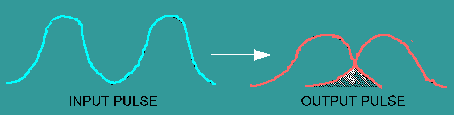MODES. - A set of guided electromagnetic waves is called the modes of an optical fiber. Maxwell's equations describe electromagnetic waves or modes as having two components. The two components are the electric field, E(x, y, z), and the magnetic field, H(x, y, z). The electric field, E, and the magnetic field, H, are at right angles to each other. Modes traveling in an optical fiber are said to be transverse. The transverse modes, shown in figure 2-17, propagate along the axis of the fiber. The mode field patterns shown in figure 2-17 are said to be transverse electric (TE). In TE modes, the electric field is perpendicular to the direction of propagation. The magnetic field is in the direction of propagation. Another type of transverse mode is the transverse magnetic (TM) mode. TM modes are opposite to TE modes. In TM modes, the magnetic field is perpendicular to the direction of propagation. The electric field is in the direction of propagation. Figure 2-17 shows only TE modes. Figure 2-17. - Transverse electric (TE) mode field patterns.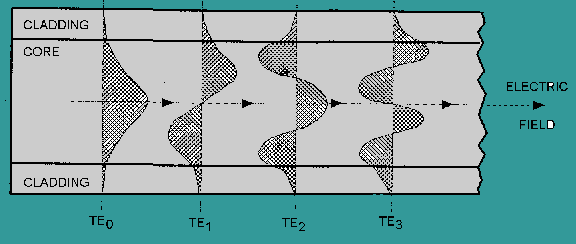The TE mode field patterns shown in figure 2-17 indicate the order of each mode. The order of each mode is indicated by the number of field maxima within the core of the fiber. For example, TE0 has one field maxima. The electric field is a maximum at the center of the waveguide and decays toward the core-cladding boundary. TE0 is considered the fundamental mode or the lowest order standing wave. As the number of field maxima increases, the order of the mode is higher. Generally, modes with more than a few (5-10) field maxima are referred to as high-order modes. The order of the mode is also determined by the angle the wavefront makes with the axis of the fiber. Figure 2-18 illustrates light rays as they travel down the fiber. These light rays indicate the direction of the wavefronts. High-order modes cross the axis of the fiber at steeper angles. Low-order and high-order modes are shown in figure 2-18. Figure 2-18. - Low-order and high-order modes.Before we progress, let us refer back to figure 2-17. Notice that the modes are not confined to the core of the fiber. The modes extend partially into the cladding material. Low-order modes penetrate the cladding only slightly. In low-order modes, the electric and magnetic fields are concentrated near the center of the fiber. However, high-order modes penetrate further into the cladding material. In high-order modes, the electrical and magnetic fields are distributed more toward the outer edges of the fiber. This penetration of low-order and high-order modes into the cladding region indicates that some portion is refracted out of the core. The refracted modes may become trapped in the cladding due to the dimension of the cladding region. The modes trapped in the cladding region are called cladding modes. As the core and the cladding modes travel along the fiber, mode coupling occurs. Mode coupling is the exchange of power between two modes. Mode coupling to the cladding results in the loss of power from the core modes. In addition to bound and refracted modes, there are leaky modes. Leaky modes are similar to leaky rays. Leaky modes lose power as they propagate along the fiber. For a mode to remain within the core, the mode must meet certain boundary conditions. A mode remains bound if the propagation constant β meets the following boundary condition: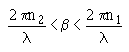where n1 and n2 are the index of refraction for the core and the cladding, respectively. When the propagation constant becomes smaller than 2πn2/λ, power leaks out of the core and into the cladding. Generally, modes leaked into the cladding are lost in a few centimeters. However, leaky modes can carry a large amount of power in short fibers. NORMALIZED FREQUENCY. - Electromagnetic waves bound to an optical fiber are described by the fiber's normalized frequency. The normalized frequency determines how many modes a fiber can support. Normalized frequency is a dimensionless quantity. Normalized frequency is also related to the fiber's cutoff wavelength. Normalized frequency (V) is defined as: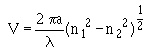where n1 is the core index of refraction, n2 is the cladding index of refraction, a is the core diameter, and λ is the wavelength of light in air. The number of modes that can exist in a fiber is a function of V. As the value of V increases, the number of modes supported by the fiber increases. Optical fibers, single mode and multimode, can support a different number of modes. The number of modes supported by single mode and multimode fiber types is discussed later in this chapter. Q.27 A light wave can be represented as a plane wave. What three properties of light propagation describe a plane wave? Q.28 A wavefront undergoes a phase change as it travels along the fiber. If the wavefront transverses the fiber twice and is reflected twice and the total phase change is equal to 1/2π, will the wavefront disappear? If yes, why? Q.29 Modes that are bound at one wavelength may not exist at longer wavelengths. What is the wavelength at which a mode ceases to be bound called? Q.30 What type of optical fiber operates below the cutoff wavelength? Q.31 Low-order and high-order modes propagate along an optical fiber. How are modes determined to be low-order or high-order modes? Q.32 As the core and cladding modes travel along the fiber, mode coupling occurs. What is mode coupling? Q.33 The fiber's normalized frequency (V) determines how many modes a fiber can support. As the value of V increases, will the number of modes supported by the fiber increase or decrease?Integrated Publishing, Inc. - A (SDVOSB) Service Disabled Veteran Owned Small Business